Next: Learning Module Exercise Up: Input Capture Interrupts Previous: Receiver

## Simplified Serial Communication Protocol

A protocol is an agreement between two parties about how the two parties should behave. A communication protocol is a protocol about how two parties should speak to each other. Serial communication protocols assume that bits are transmitted in series down a single channel. A serial protocol has to address the following issues

• How does the receiver know when to start looking for information?
• When should the receiver look at the channel for the information bits?
• What is the bit order? (MSB or LSB first)
• How does the receiver know when the transmission is complete?

The IR link you'll be constructing is an asynchronous serial link and this means that these four issues must be agreed upon by both transmitter and receiver prior to sending any information.

In many serial protocols, the issue of how to detect the start of a transmission uses a special bit known as a start bit. Both transmitter and receiver assume that the channel is initially idle (i.e. zero volt logical level). A transmission is then signalled by setting the channel high for a specified length of time and then setting it low again. Transmission of information bits usually commences a specified interval of time after the falling edge of the start bit.

The issue of "when" to look for data bits must be agreed upon prior to establishing the link. Asynchronous serial protocols usually require that information bits be transmitted at regular time intervals. For instance if we have a 2400 kbaud modem, then both receiver and transmitter know that they should look for information bits arriving at a rate of 2400 thousand bits per second.

One possible way of formulating the protocol is to assume that the start bit is identified by a rising edge. Let's assume that the information and start bits are being transmitted at a rate of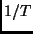bits per second. The the first information bit will occur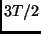seconds after the start bit's rising edge, and then every succeeding information bit can be read every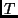seconds after the last bit was read. Figure 53 illustrates the proposed format for the serial frame of data.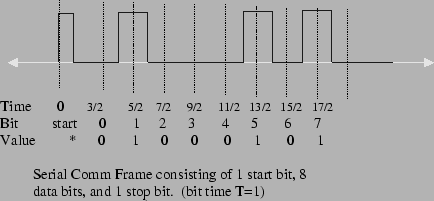Once you've recovered a series of bits, there is a natural question concerning the order of the transmitted bits. In other words if each transmission consists of a byte, then is the first bit received the most or least significant bit in that byte. The receiver and transmitter need to agree upon the bit order prior to transmission so the bits are properly interpreted.

Determining when a transmission should end can again use something like a stop bit of durationseconds to indicate the end of transmission. The stop bit makes sure that there is at least T seconds between the last information bit and the start bit of the next transmitted byte.

To summarize, the comm-protocol we advise you using in this learning module consists of the following agreements. In this protocol, the variableis a bit time interval agreed upon by the receiver and transmitter. We'll assume thatequals 10 milliseconds.

• The transmitter signals the start of a transmission by setting the channel high for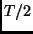seconds and then low forseconds . We'll refer to this pulse as the start-bit.
• The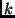th information bit's rising edge occurs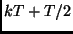seconds after the falling edge of the start-bit.
• Each transmission consists of one start bit and exactly 8 information bits (i.e.,ranges between 0 and 7).
• After the transmission is completed, then we'll use one stop-bit of duration T seconds.
• The first information bit after the start-bit is the LSB of the transmitted byte.
The timing diagram for this serial protocol is shown in figure 53. The protocol itemized above is only a recommendation, a starting point. Feel free to change it if necessary.Next: Learning Module Exercise Up: Input Capture Interrupts Previous: Receiver
Bill Goodwine 2002-09-29